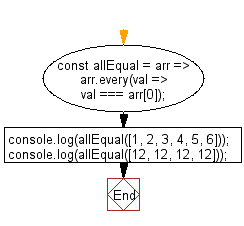# JavaScript: Check whether all elements in a given array are equal or not

## JavaScript fundamental (ES6 Syntax): Exercise-56 with Solution

Write a JavaScript program to check whether all elements in a given array are equal or not.

• Use Array.prototype.every() to check if all the elements of the array are the same as the first one.
• Elements in the array are compared using the strict comparison operator, which does not account for NaN self-inequality.

Sample Solution:

JavaScript Code:

``````//#Source https://bit.ly/2neWfJ2
const allEqual = arr => arr.every(val => val === arr);
console.log(allEqual([1, 2, 3, 4, 5, 6]));
console.log(allEqual([12, 12, 12, 12]));
```
```

Sample Output:

```false
true
```

Pictorial Presentation:Flowchart:Live Demo:

See the Pen javascript-basic-exercise-56-1 by w3resource (@w3resource) on CodePen.

Improve this sample solution and post your code through Disqus

What is the difficulty level of this exercise?

Test your Programming skills with w3resource's quiz.

﻿

## JavaScript: Tips of the Day

Chunks an array into n smaller arrays

Example:

```const tips_chunkIntoN = (arr, n) => {
const size = Math.ceil(arr.length / n);
return Array.from({ length: n }, (v, i) =>
arr.slice(i * size, i * size + size)
);
}
console.log(tips_chunkIntoN([1, 2, 3, 4, 5, 6, 7,8], 4));
```

Output:

```[[1,2],[3,4],[5,6],[7,8]]
```